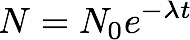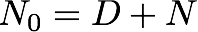## Sunday, April 22, 2007

### The Age Equation

This post is inspired by some emails I've received as well as a good group of google searches that have pointed folks to Apparent Dip. The Age Equation is central to geochronology, and forms the basis for age calculations. It is actually fairly simple to derive, so here we go

In the following derivation, N is the number of radioactive parent atoms at time t, N0 is the original number of radioactive parent atoms, D is the number of stable daughter atoms, λ is the decay constant, and t½ is the half life of a radioactive atom. The situation I use as an example is one parent and one stable daughter. Slight modifications are needed for multiple parents (the (U-Th)/He system), multiple daughters (40K decay), or the use of unstable daughters in a decay chain (U-series dating).

The activity, or rate of decay of a radioactive isotope is proportional to the number of radioactive atoms presentBy introducing a proportionality constant λ (which we will call the decay constant), we can turn the above equation intoBecause the λ is in units time-1, and the number of atoms decreases with time, we also throw in a negative sign. Rearrange this equationand integrate from N0 (original number of radioactive parent atoms) and t=0 (time zero)and we getsince t0 = 0, this leads towhich can be rearranged toThe above equation describes the number of radioactive atoms left after time t. The decay constants, along with their uncertainties, are readily available (I'll list some of the geologically important ones at the end of this post). Decay constants are inherent properties of the nucleus that describe the probability that an atom will decay. I blogged about demonstrating this phenomenon with M&M's here

OK, so the above equation is handy, but when we work with geologic samples, we don't know N0, the original number of parent atoms in the crystal, so can we put this equation into terms of things we can measure today?

In a system with one parent and one daughter, N0 is the sum of N, the number of parents left, and D, the number of daughters produced.So if we put the last two equations together, we get thisWhich is entirely in terms of things we can measure in the lab. The next steps are simple mathematical rearrangementsUntil we get an equation that solves for time and whose variables are all things that we can measure.I've been using the decay constant up until now, which as I said earlier describes the probability that an atom will decay. The half-life of an isotope is a more intuitive measure, the half-life is a unit of time over which half of the radioactive atoms in a given population will decay. You can easily see the relationship between the decay constant (λ) and half-life (t½) with this simple exercise. At t½, half of the parents have decayed and turned into daughters, so N=D, and D/N = 1. So let's plug that into our age equationsand we get the relationship between λ and t½So the equations are pretty straightforward. As I said, they get a bit more complicated for more complicated decay schemes, but the basics are all the same.

Here are some of the more important radioactive isotopes, decay constants, and half lives. I got this information from table 1.1 of Alan Dickin's Radiogenic Isotope Geology (2ed), he has the references for the appropriate scientific papers listed as well.

 Isotope Decay Constant (yr-1) Half-Life 40K (40Ar) 5.81 X 10-11 11.93 Ga 40K (40Ca) 4.962 X 10-10 1.397 Ga 40K (total) 5.543 X 10-10 1.25 Ga 235U 9.8485 X 10-10 703.8 Ma 238U 1.55125 X 10-10 4.468 Ga 232Th 4.9475 X 10-11 14.01 Ga 87Rb 1.402 X 10-11 49.44 Ga 147Sm 6.54 X 10-12 106 Ga 176Lu 1.86 X 10-11 37.3 Ga

And, as usual Ga means billion years, and Ma is million years. Now you too can enjoy hittin' the geochronic.

C W Magee said...

87Sr is the stable daughter. I think you want 87Rb listed as the parent.

Also, it is important to note that geologists use YEARS to denote time (either lambda or halflife), while physicists often list decay constants in 1/SECONDS. Failing to recognize this difference will give an answer that is wrong by a factor of 31 million.

Thermochronic said...

Nice eye, that post went from publich to error checking in less than 15 minutes! And yes, this blog is geocentric (no offense to THM, definite intentional offense to physics man).

Thermochronic said...

Ha! What the hell is publich! Doesn't this idiot blogger mean publish?Anonymous said...

I'm too many years away from the math to check the calculations, but you might want to check your spelling of derivation in the first line of your second paragraph.

Thermochronic said...

My blogging skills have obviously become rusty during my recent lull, who says there is no peer review on the web?

BrianR said...

who said blogging isn't rigorous...the commenters are keepin' you honest!

nice post

Don Thieme said...

Excellent summary of isotopic dating. I will no doubt be making use of this in the future.

Thermochronic said...

Thanks, I appreciate the comment! Whenever I have taught geochronology to any group of students, I've found that it helps not to treat the process as a black box. The governing principles and equations are really not that difficult, and when they see that they can figure things out on their own they feel very empowered.

Don Thieme said...

I have a simple homework exercise posted here:

http://www.gpc.edu/~dthieme/Homework/1122-Homework1.htm

It really leaves the use of "ln 2/lambda" for the half-life a mystery, however. The students do see the reciprocal relationship. I plan not only to add more problems on isotopes, but also to put together a general tutorial on exponential growth and decay.